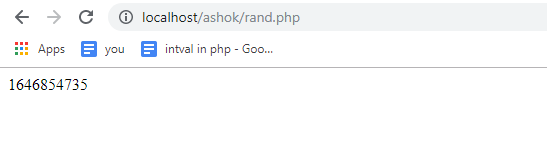AWS Global Infrastructure

### Programming & Frameworks

Topics Covered
• C Programming and Data Structures (16 Blogs)
• Comprehensive Java Course (4 Blogs)
• Java/J2EE and SOA (346 Blogs)
• Spring Framework (8 Blogs)
SEE MORE

# How to Implement a Random Number Generator in PHPAt some point, when you program in PHP, you would need to generate random numbers. In this article, we will see how to generate a random number and also we will see how to specify a lower and an upper limit with a random number generator in PHP. The following pointers are covered in this article:

## rand() method for Random Number Generation

When we use the rand(), we can either supply no arguments at all or we can supply 2 arguments. Firstly, we will look at supplying no arguments. So if I wish to just echo round() or in fact create a variable called rand that we can use later in our program. The random number in PHP is automatically seeded. so there is no need to do anything at that moment because we have not supplied our arguments. This is going to generate a random number between a minimum and maximum amount. The minimum amount is 0 or 1 and the maximum is a prespecified amount.

```<?php
\$rand=rand();
echo \$rand; ?>
```It generates a random number every time when we refresh the browser

There is also a way where we can find out the upper value so let’s understand by coding it out

```<?php
\$rand=rand();
\$max=getrandmax();
echo \$rand. '/'.\$max; ?>
```Moving on with this article on random number generator in PHP.

## getrandmax()

So getrandmax() will return the largest possible random value that can be returned by rand() essentially what we are seeing is that random number out of the maximum. So 2147483647 is the maximum number in this case that can be generated and the number that has been generated is 511779142.

As we refresh, the maximum value will be same and however, the random number generated will change.Let see if you want to create a dice game where the user has to roll a dice randomly.

```<?php
if(isset(\$_POST['roll']))
{
\$rand=rand(1,6);
echo 'Dice shows'." ".\$rand;
}
?>
<form action="rand.php" method="POST">
<input type="submit" name="roll" value="roll dice">
</form>
```Every time you click on roll dice, it generates a random number. With this, we come to an end of this Random Number Generator in PHP article. I hope you have learned how to generate random number in PHP and the use case of generating the random number.

check out the PHP Certification Training by Edureka, a trusted online learning company with a network of more than 250,000 satisfied learners spread across the globe.

Got a question for us? Please mention it in the comments section of ”Random Number Generator in PHP” and I will get back to you.REGISTER FOR FREE WEBINARThank you for registering Join Edureka Meetup community for 100+ Free Webinars each month